# Introduction

Graphs have taken a lot of attention during the last years, from graph machine learning methods, including Graph Neural Networks, to Graph Databases. Even on Medium, people are posting graph articles more than before. One thing that I believe most data scientists underestimates is the power of graphs in visualization and storytelling.

Many see graphs as a complex and expensive solution for modeling data science problems. However, they still provide a great way to visualize data that no other chart or visualization can.

In this blog post, I will use the Mathematicians of Wikipedia dataset to investigate relations between mathematicians and their advisors using NetworkX to analyze and visualize.

# What is NetworkX?

NetworkX is one of the most popular frameworks to work with. I think it is the scikit-learn of graph world! It is most probable to find NetworkX sooner than any other graph library when you start to learn about graphs and graph frameworks.

NetworkX provides many graph implementations, algorithms, and methods of analysis. Even though it is slow for many real applications, it still offers great functionalities worth learning.

# Understaning the data

I have found the dataset from Kaggle. The table below demonstrates all the available features for this dataset that describes mathematicians. Note that the examples do not come from the same row.

The highlighted column names ( `mathematicians` and `doctoral advisor`) are the columns in which I am interested in analyzing and showing how mathematicians are connected. So then, my first step is to load the dataset and clean it.

`import pandas as pd import networkx as nx import matplotlib.pyplot as plt import numpy as np df = pd.read_csv('../data/data_cleaned.csv')`

The first problem with the data is that many of its columns, including `doctoral advisor`, are a list of names embedded as a string. As I am only interested in the `doctoral advisor` column, I will only fix this column, but you can add the column names to the `list_type_columns` to fix it as well.

`list_type_columns = [    'doctoral advisor']df[list_type_columns] = df[list_type_columns].fillna('[]')for column in list_type_columns:    df[column] = df[column].str.replace("'", '', regex=False)    df[column] = df[column].str.replace("[", '', regex=False)    df[column] = df[column].str.replace("]", '', regex=False)    df[column] = df[column].str.split(',')df = df.explode(column)`

Now that I have handled my dataset issues, including Null values, by replacing them with empty lists and fixing the string embedded list to real lists, I can save it to a CSV file to use later, so I won’t need to process my dataset every time.

`df.dropna(inplace=True)df[['mathematicians', 'doctoral advisor']].to_csv('../data/adv.csv', index=False)`

I only saved the columns I needed, including `mathematicians` and `doctoral advisor`.

# PageRank Algorithm

PageRank computes the rank of each node based on the number of incoming edges. Google initially developed PageRank to calculate the importance of web pages.

PageRank works by counting the number and quality of links to a page to determine a rough estimate of how important the website is. The underlying assumption is that more important websites are likely to receive more links from other websites.

Using RankPage in NetworkX is really easy:

`G = nx.DiGraph(nx.path_graph(4)) pr = nx.pagerank(G, alpha=0.9)`

# Graph Visualization has more insights

Now that we have loaded our dataset, cleaned it, and fixed its issues, let’s see the top mathematicians with the most students.

`top_ten_advisor = df['doctoral advisor'].value_counts().sort_values(ascending=False).head(15)fig, ax = plt.subplots()ax.barh(top_ten_advisor.index, top_ten_advisor.values)ax.set_xlabel('Number of Students')ax.axvline(x=13, c='red')plt.show()`

output:

Even though the above image shows the most famous mathematicians and their students, it does not describe how they are connected and leaves lots of questions unanswered:

1. Are these top advisors connected by a shared student?
3. Do students also advise other students?

To address the questions above, a graph representation can help us. First, let’s drop all the advisors with less than 13 students.

`minimum_count = 13df = df[df['doctoral advisor'].isin(df['doctoral advisor'].value_counts()[df['doctoral advisor'].value_counts() >= minimum_count].index)]`

We create our graph in `NetworkX` and calculate the PageRanks ratings.

`graph = nx.DiGraph()graph.add_nodes_from(np.unique(df.values.flatten()))graph.add_edges_from(df.values)pr = nx.pagerank(graph)names, ranks = zip(*pr.items())pr_df = pd.DataFrame(data={'mathematicians': names, 'rank': ranks})pr_df`

Now that we have both graphs and ranks, we can draw.

`fig = plt.figure(1, figsize=(30, 20), dpi=100)pos = nx.spring_layout(graph, k=1.1*1/np.sqrt(len(graph.nodes())), iterations=20)nx.draw(graph, node_size=pr_df['rank'].values*10000, with_labels=True, pos=pos, edge_color='gray')plt.show()`

Originally published at https://kiani.info.

--

--

--

## More from Kiarash Kiani

Machine Learning Engineer, kiani.info

Love podcasts or audiobooks? Learn on the go with our new app.

## Union-Find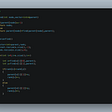## If you don't know what hunting is,then read this.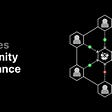## Basics of Statistics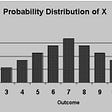## Data Integrity: How do we identify irregularities across multiple sources of data?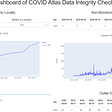## Skymatics Puts Computer Vision to Work, Providing Insights into the Causes of Crop Damage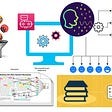## Is Pentaho Data Integration a low hanging fruit to be grabbed## Predicting Snowmelt Patterns with Deep Learning and Satellite Imagery## Kiarash Kiani

Machine Learning Engineer, kiani.info

## Speech recognition and text reinterpretation for clear and error-proof text## “combines CPU and GPU cores onto a single package for data centers and AI” revolutionizing…## Challenges facing a computational biologist in a core facility## What Is Concept Drift And Why Does It Go Undetected?